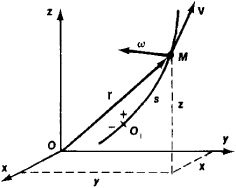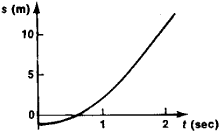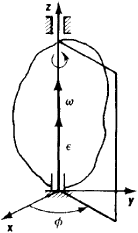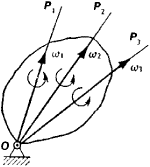# kinematics

(redirected from Cinematics)
Also found in: Dictionary, Thesaurus, Medical, Wikipedia.

## kinematics:

see dynamicsdynamics,
branch of mechanics that deals with the motion of objects; it may be further divided into kinematics, the study of motion without regard to the forces producing it, and kinetics, the study of the forces that produce or change motion.
..... Click the link for more information.
.
The Columbia Electronic Encyclopedia™ Copyright © 2013, Columbia University Press. Licensed from Columbia University Press. All rights reserved. www.cc.columbia.edu/cu/cup/

## Kinematics

That branch of mechanics which deals with the motion of a system of material particles without reference to the forces which act on the system. Kinematics differs from dynamics in that the latter takes these forces into account. See Dynamics

For a single particle moving in a straight line (rectilinear motion), the motion is prescribed when the position of the particle is known as a function of the time. Plane kinematics of a particle is concerned with the specification of the position of a particle moving in a plane by means of two independent variables. The kinematics of a particle in space is concerned with the ways in which three independent coordinates may be chosen to specify the position of the particle at a given time, and with the relations between the first and second time derivatives of these coordinates and the components of velocity and acceleration of the particle.

Among the coordinate systems studied in kinematics are those used by observers who are in relative motion. In nonrelativistic kinematics the time coordinate for each such observer is assumed to be the same, but in relativistic kinematics proper account must be taken of the fact that lengths and time intervals appear different to observers moving relative to each other. See Relativity

McGraw-Hill Concise Encyclopedia of Physics. © 2002 by The McGraw-Hill Companies, Inc.
The following article is from The Great Soviet Encyclopedia (1979). It might be outdated or ideologically biased.

## Kinematics

a branch of mechanics dealing with the study of geometric properties of the motion of bodies, without taking into account their masses or forces acting on them. The discussion that follows refers to the kinematics of motions treated in classical mechanics (motions of macroscopic bodies at velocities that are low in comparison with speed of light).

The methods and relationships established in kinematics are applied in the kinematic study of motions, particularly in calculations of the transmission of motion in various mechanisms and machines, as well as in solving problems of dynamics. Kinematics is subdivided into kinematics of particles, kinematics of rigid bodies, and kinematics of a continuous variable medium (a deformable body, a fluid, or a gas), depending on the properties of the object being studied.

In kinematics the motion of any object is studied with reference to some other body (a reference body), with which a frame of reference is associated (the axes x, y, and z in Figure 1). The frame of reference is used to define the position of the moving body relative to the reference body at various moments. The choice of a frame of reference is arbitrary and depends on the purpose of the research. For example, in studying the motion of a wheel of a railroad car relative to the rail the frame of reference is associated with the ground. In studying the motion of the same wheel relative to the body of the car, the frame of reference is associated with the body. The motion of the object under study is considered to be specified (known) if certain equations—the equations of motion (or graphs or tables)—are known; such equations define the position of the object relative to the frame of reference at any moment. The main problem in kinematics is the determination (with the aid of a particular mathematical method) of a means of specifying the motion of points or bodies and the determination from their equations of motion of the corresponding kinematic characteristics, such as trajectory, velocity, and acceleration of moving points and angular velocity and angular acceleration of rotating bodies. Three methods (the natural, coordinate, and vector methods) are used to specify the motion of a point.Figure 1

The natural (or trajectory) method is used if the trajectory of a point relative to the chosen frame of reference is known. The position of the point is determined by the distance s = O1M from the chosen point of origin O1 on the trajectory; the distance is measured along the arc of the trajectory and is used with the appropriate sign (see Figure 1). The law of motion is given by the equation s = f(t) which describes the dependence of s on time t. For example, if s = 3t2 – 1, then at the initial moment t0 = 0, s0 = – 1 m (a point located 1 m to the left of the origin O1). At t1 = 1 sec, s1 = 2m (a point 2 m to the right of O1), and so on. The dependence of s on t can also be represented by a motion diagram, in which time is plotted in a chosen scale along the t axis and distance is plotted along the s axis (see Figure 2), or by a table, where the values of t are given in one column and the corresponding values of s in another column (this method is used in railroad schedules).Figure 2

In the coordinate method, the position of a point relative to the frame of reference is defined by any three coordinates—for example, by the rectangular Cartesian coordinates x, y, and z. The law of motion is given by three equations x = f1(t), y = f2(t) and z = f3(t). The trajectory of a point may be found by eliminating the time t from these equations.

In the vector method, the position of a point relative to the frame of reference is determined by the radius vector r, drawn from the point of origin to the moving point. The law of motion is given by the vector equation r = r (t). The trajectory of the point is the hodograph of the vector r.

The basic kinematic characteristics of a moving point are its velocity and acceleration, the values of which are determined by equations of motion, using first and second derivatives with respect to time of s, of x, y, and z, or of r.

The methods for specifying the motion of a rigid body depend on the type of its motion. The number of equations of motion depends on the number of degrees of freedom of the body. The simplest motions of a rigid body are translation and rotation. In translation all points of a body move identically; its motion is formulated and studied in the same way as the motion of one point. In rotation around a fixed axis z (Figure 3), the body has one degree of freedom; its position is determined by the angle of rotation ϕ, and the law of motion is given by the equation ϕ = f(t). The basic kinematic characteristics of the body are its angular velocity ѡ = dϕ/dt and angular acceleration = dѡ/dt The quantities ѡ and are depicted as vectors directed along the axis of rotation. If ѡ and ѡ are known, the velocity and acceleration of any point of the body may be determined.Figure 3

The motion of a body having only one fixed point and three degrees of freedom—for example, a gyroscope or a top—is more complex. In this case a position of the body relative to the frame of reference is determined by any three angles (for example, the Eulerian angles: precession, nutation, and spin). The law of motion is determined by equations that describe the dependence of the angles on time. The basic kinematic characteristics of a body are the instantaneous angular velocity ѡ and the instantaneous angular acceleration ɛ. The motion of the body involves a series of elementary turns around instantaneous rotational axes OP, which pass through the fixed point O (Figure 4) and continuously change their direction.Figure 4

The most general case is that of the motion of a free rigid body having six degrees of freedom. The position of the body is determined by three coordinates of one of its points, called the pole (in problems of dynamics the center of gravity is assumed to be the pole), and by three angles chosen in the same way as for a body with a fixed point. The law of motion for the body is given by six equations that describe the dependence of the coordinates and angles on time. The motion of the body involves translation along the pole and a rotation around the pole, in the same way as around a fixed point. Examples of such motion are the motion of an artillery projectile, the motion of an airplane engaged in aerobatics, and the motion of heavenly bodies. The basic kinematic characteristics of a body are its velocity and acceleration of the translational part of the motion, which are equal to the velocity and acceleration of the pole, and angular velocity and acceleration of the rotation of the body around the pole. All these characteristics, like the kinematic characteristics for a body with a fixed point, are computed from the equations of motion; if the characteristics are known, the velocity and acceleration of any point of the body may be determined. A special case of the motion being considered is the plane motion of a rigid body, in which all of its points move parallel to a plane. Such motion is performed by components of many mechanisms and machines.

The compound motion of points and bodies—that is, motion examined simultaneously with respect to two or more frames of reference that are moving relative to each other—is also studied in kinematics. In this case one of the frames of reference is regarded as the system of absolute coordinates (also called the conventionally fixed system), and the frame of reference moving with respect to the primary system is called the system of relative coordinates. In the general case there may be several systems of relative coordinates.

In studying compound motion of a point, its motion—as well as its velocity and acceleration—is called conventionally absolute with respect to the system of absolute coordinates and conventionally relative with respect to the system of relative coordinates. The motion of the system of relative coordinates itself and of all points of space physically connected to it is called the motion of transport, and the velocity and acceleration of the point of the system of relative coordinates with which the moving point coincides at a given moment are called the velocity and acceleration of following. For example, if the system of absolute coordinates is connected with a shore and the system of relative coordinates is connected with a steamship moving on a river, and if the rolling of a ball on the deck of the ship is considered (the ball is taken to be a point), then the velocity and acceleration of the ball with respect to the deck are relative, whereas those with respect to the shore are absolute. However, the velocity and acceleration of a point of the deck touched by the ball at a given moment are the transport characteristics for the ball. Analogous terminology is used in studying compound motion of rigid bodies.

The main problems in kinematics of compound motions are the determination of the relations between the kinematic characteristics of the absolute and relative motions of a point (or a body) and the motion characteristics of the system of relative coordinates (that is, the motion of transport). For a point the corresponding equations are as follows: the absolute velocity of a point is equal to the vector sum of the relative velocity and the transport velocity,

Vabs = Vrel + Vtr

and the absolute acceleration of a point is equal to the vector sum of three accelerations (the relative, transport, and rotational, or Coriolis),

Wabs = Wrel + Wtr + Wcor

For a rigid body, when all the component motions (that is, the relative motion and the motion of transport) are translational the absolute motion is also translational, with a velocity equal to the sum of the velocities of the component motions. If the component motions of a body are those of rotation around axes intersecting at one point (as in a gyroscope), then the resultant motion will also be rotation around the point, with an instantaneous angular velocity equal to the vector sum of the angular velocities of the component motions. If the component motions of a body are both translational and rotational, then the resultant motion will usually consist of a series of instantaneous helical motions.

The kinematics of a continuous medium establishes methods for formulating a specifying the motion of such a medium, studies the general theory of deformation, and gives the continuity equations, which reflect the conditions for continuity in a medium.

S. M. TARG

The Great Soviet Encyclopedia, 3rd Edition (1970-1979). © 2010 The Gale Group, Inc. All rights reserved.

## kinematics

[¦kin·ə¦mad·iks]
(mechanics)
The study of the motion of a system of material particles without reference to the forces which act on the system.
McGraw-Hill Dictionary of Scientific & Technical Terms, 6E, Copyright © 2003 by The McGraw-Hill Companies, Inc.

## kinematics

the study of the motion of bodies without reference to mass or force
Collins Discovery Encyclopedia, 1st edition © HarperCollins Publishers 2005
References in periodicals archive ?
Together with Joachim Tobias, he formed the house and broken beat project Inverse Cinematics and many remixes and compilation appearances followed.
Through this acquisition AL acquires MEL's animation and visual effects education brand Maya Academy of Advanced Cinematics (MAAC).
Within sections on Deleuzian sciences, sociotechnical becomings, and minor assemblages, they consider experimenting with What is Philosophy, irony and humor, sub-representative differences in digital video codecs, cybernetics as nomad science, cinematics of scientific images, and intensive filiation and demonic alliance.
With absolutley stunning cinematics and cut scenes, I was hooked immediately from the intro.
John Kelly THE CINEMATICS King Tut's, Glasgow, April 20 The Glasgow four-piece did little to boost their following with a collection of unremarkable songs.
In addition, live-action cinematics also return with performances from Jamie Chung, 16-time world heavyweight wrestling champion, 'Nature Boy' Ric Flair, Malcolm McDowell and Holly Valance, alongside with favourites, Gemma Atkinson and Ivana Milicevic.
In Cinematica (O hipnotizador de troncos) (Cinematics [The Log Hypnotizer]), 2006, a man reveals the special effect that allows him to appear to be piling up tree trunks using only the power of his mind, and in A coluna de Colombo (The Columbus Column), 2006, a man stacks eggs, an allusion to the popular term "egg of Columbus," meaning something that is obvious once it has been demonstrated.
The End of Cinematics is one of those shows that you are unlikely to forget.
The 3ds max 7.5 software subscription upgrade will especially equip 3ds max software artists working in feature film and game cinematics who want to raise the bar for their 3ds max software work with amazingly realistic hair and fur.
"Una Bella Pizza" and "Making A Fine Bouillabaisse" are both reproduced as full-size 3-D Cinematics, with image sizes of 24 by 19 inches and 25 1/4 by 19 inches respectively.
The trilogy also includes enhanced environments, a remastered soundtrack, updated controls, brand-new lighting and recreated cinematics for added pizzazz in this beloved adventure.
Alerion owns the intellectual property and other assets relating to unmanned aerial systems developed under the program name "Aerial Cinematics." Aerial Cinematics is an Unmanned Aerial Systems based photography, mapping and surveillance systems design project.

Site: Follow: Share:
Open / Close